Click to Chat

1800-1023-196

+91-120-4616500

CART 0

• 0

MY CART (5)

Use Coupon: CART20 and get 20% off on all online Study Material

ITEM
DETAILS
MRP
DISCOUNT
FINAL PRICE
Total Price: Rs.

There are no items in this cart.
Continue Shopping```Elementary Operations of a MatrixThe following three operations applied on the rows (columns) of a matrix are called elementary row (column) transformations.

(i) Interchange of any two rows (columns)

If ith row (column) of a matrix is interchanged with the jth row (column), it will be denoted by Ri ↔ Rj (Ci ↔ Cj).

For example: A =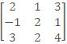, then by applying R1 → R2 we get B =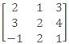.

(ii) Multiplying all elements of a row (column) of a matrix by a non-zero scalar. If the elements of ith row (column) are multiplied by non-zero scalar k, it will be denoted by Rl→Ri (k) [Ci→Ci (k)] or Rl→kRi [Ci→kCi].

If A =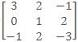, then by applying R2 → 3R2, we obtain B =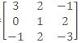.

(iii) Adding to the elements of a row (column), the corresponding elements of any other row (column) multiplied by any scalar k.

If k times the elements of jth row (column) are added to the corresponding elements of the ith row (column), it will be denoted by Ri→Ri + k Rj (Ci→Ci + kCj).

If A=, then application of elementary operation R3→R3 + 2R1 lead to B =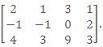.

Illustration:

Using elementary row transformations and find the inverse of the matrix A=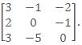.

Solution: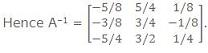IIT JEE and AIEEE study material is available online free of cost at askIITians.com. We offer numerous live online courses, online tests and other interesting sections as well for IIT JEE and AIEEE preparation - you do not need to travel anywhere any longer - just sit at your home and study for IIT JEE and AIEEE live online with askIITians.com

To read more, Buy study materials of Matrices and Determinants comprising study notes, revision notes, video lectures, previous year solved questions etc. Also browse for more study materials on Mathematics here.
```### Course Features

• 731 Video Lectures
• Revision Notes
• Previous Year Papers
• Mind Map
• Study Planner
• NCERT Solutions
• Discussion Forum
• Test paper with Video Solution